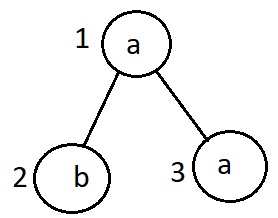Nodes in a subtree
Tag(s):

## Binary/ N-ary Trees, Data Structures, Depth-first search, Easy, Hash function, Tree, Trees

Problem
Editorial
Analytics

You are given a rooted tree that contains $N$ nodes. Each node contains a lowercase alphabet.

You are required to answer $Q$ queries of type $u, c$, where $u$ is an integer and $c$ is a lowercase alphabet. The count of nodes in the subtree of the node $u$ containing $c$ is considered as the answer of all the queries.

Input format

• First line: Two space-separated integers $N\ and\ Q$ respectively
• Second line: A string $s$ of length $N$ (where the $i^{th}$ character of $s$ represents the character stored in node $i$)
• Next $N - 1$ line: Two space-separated integers $u$ and $v$ denoting an edge between node $u$ and node $v$
• Next $Q$ lines: An integer $u$ and a space-separated character $c$

Output format
For each query, print the output in a new line.

Constraints

$1 \leq N, Q \leq 10^5\\ 1 \leq u, v \leq N$

• $c$ is a lowercase alphabet
• $s_i$ is a lowercase alphabet for all $1 \leq i \leq N$
• $1$ is the root node

Note: It is guaranteed that the input generates a valid tree.

SAMPLE INPUT
3 1
aba
1 2
1 3
1 a
SAMPLE OUTPUT
2

Explanation

Tree given in the sample input will look like that.Number of nodes in the subtree of node 1 having 'a' stored in it is 2.

Time Limit: 1.0 sec(s) for each input file.
Memory Limit: 256 MB
Source Limit: 1024 KB
Marking Scheme: Marks are awarded when all the testcases pass.
Allowed Languages: Bash, C, C++, C++14, Clojure, C#, D, Erlang, F#, Go, Groovy, Haskell, Java, Java 8, JavaScript(Rhino), JavaScript(Node.js), Julia, Kotlin, Lisp, Lisp (SBCL), Lua, Objective-C, OCaml, Octave, Pascal, Perl, PHP, Python, Python 3, R(RScript), Racket, Ruby, Rust, Scala, Swift, Swift-4.1, TypeScript, Visual Basic

## CODE EDITOR

Initializing Code Editor...

## This Problem was Asked inChallenge Name

Capillary Java Hiring Challenge - June 2019

OTHER PROBLEMS OF THIS CHALLENGE
• Data Structures > Arrays